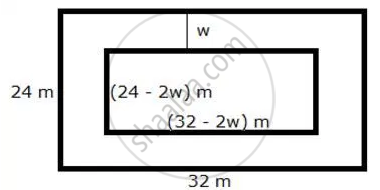Share

# A Footpath of Uniform Width Runs Round the Inside of a Rectangular Field 32 M Long and 24 M Wide. If the Path Occupies 208 M², Find the Width of the Footpath. - Mathematics

Course

#### Question

A footpath of uniform width runs round the inside of a rectangular field 32 m long and 24 m wide. If the path occupies 208 m², find the width of the footpath.

#### Solution

Let w be the width of the footpath.Area of the path = Area of outer rectangle – Area of inner rectangle
∴ 208 = (32)(24) – (32 – 2w)(24 – 2w)
208 = 768 – 768 + 64w + 48w – 4w2
4w2 – 112w + 208 = 0
w2 – 28w + 52 = 0
w2 – 26w – 2w + 52 = 0
w(w – 26) – 2(w – 26) = 0
(w – 26) (w – 2) = 0
w = 26, 2
If w = 26, then breadth of inner rectangle = (24 – 52) m = -28 m, which is not possible.
Hence, the width of the footpath is 2 m.

Is there an error in this question or solution?